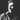# High-level Node.js streams with Highland

January 28, 2016

Node.js has a simple and powerful stream API. Streams in Node.js are unix pipes that let you perform asynchronous I/O operations by reading source data and pipe it to destination. If your application operates not with streams only, but promises, callbacks or synchronous code, you may want to use more deeper abstraction that fits your needs. In this case you may take a look at Highland.

## Highland

Highland library allows you to manage asynchronous and synchronous code easily both in Node.js and in the browser. With Highland you can simple switch between synchronous and asynchronous data sources without re-writing your code. You can install Highland with NPM:

``npm install highland``

and require or import it as yet another Node.js module:

``import \_ from "highland"``

## General examples

Converting from arrays to Highland Streams:

``\_([0, 1, 2]).toArray(xs => console.log(xs)) // 0, 1, 2``

Map and reduce over a stream:

``````\_([0, 1, 2])
.map(x => x + 1)
.reduce(1, (a, b) => a \* b); // ``````

``````import fs from "fs"

Handling errors:

``````stream.errors((err, rethrow) => {
console.error(err)
})``````

Pipe to Node.js streams:

``stream.pipe(outputStream)``

## Stream objects

Constructor:

``const stream = \_(source) // source - Array/Generator/Node Stream/Event Emitter/Promise/Iterator/Iterable``

General functions:

``````stream.destroy()
stream.end()
stream.pause()
stream.resume()
stream.write(x) // Write x value to stream``````

## Transformations

``````_([0, 1, 2]).append(3) // [0, 1, 2, 3]
_([0, 1, 2, null, undefined]).compact() // [1, 2]
_([1, 2, 5]).filter(x => x <= 3) // [1, 2]
_([1, 2, 3]).last() // 3
_(["ABC"]).invoke("toLowerCase", []) // abc
_([{ foo: "bar" }]).pick(["foo"]) // { foo: 'bar' }
_([{ foo: "bar" }]).pluck(["foo"]) // bar
_([0, 1, 2, 3]).slice(2, 4) // [2, 3]
_(["c", "a", "b"]).sort() // ['a', 'b', 'c']
\_([0, 0, 2, 3]).uniq() // [0, 2, 3]``````

## High-order Streams

``````_([0, 1]).concat([2, 3]) // [0, 1, 2, 3]
_([0, 1, [2, ]]).flatten() // [0, 1, 2, 3]
_(_([0, 1]), _([2, 3])).sequence() // [0, 1, 2, 3]
_(["a", "b"]).zip([1, 2]) // => ['a', 1], ['b', 2]

const fork = stream.fork()
const observer = stream.observe()
fork.resume()``````

## Objects

``````_.extend({ name: "foo" }, { type: "obj" }) // { name: 'foo', type: 'obj' }
_.get("foo", { foo: "bar" }) // bar
_.keys({ foo: "bar" }) // ['foo']
_.values({ foo: "bar" }) // ['bar']
\_.pairs({ foo: "bar" }) // ['foo', 'bar']``````

## Utils

``````_.isStream([1, 2, 3]) // false
_.isStream(stream) // true

_([1, 2, 3]).each(_.log) // 1, 2, 3

## Conclusion

If you need to handle your synchronous and asynchronous data with differrent abstractions in one way, operate with Node.js streams at higher level, you can use Highland high-level streams library to fit your needs. You can find more at Highland docs.

Tags:Aleksei Kuznetsov

Functional programming, software architecture, DevOps

Salam